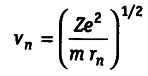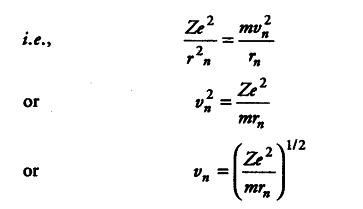# For a hydrogen-like particle, derive the expression

For a hydrogen-like particle, derive the expressionwhere { v }_{ n } is the velocity of the electron at distance r„ from the nucleus, Z is the atomic number of the H-like particle, m and e are the charge and mass of the electron.

For H-Iike particle, force of attraction between the electron and the nucleus = centrifugal force